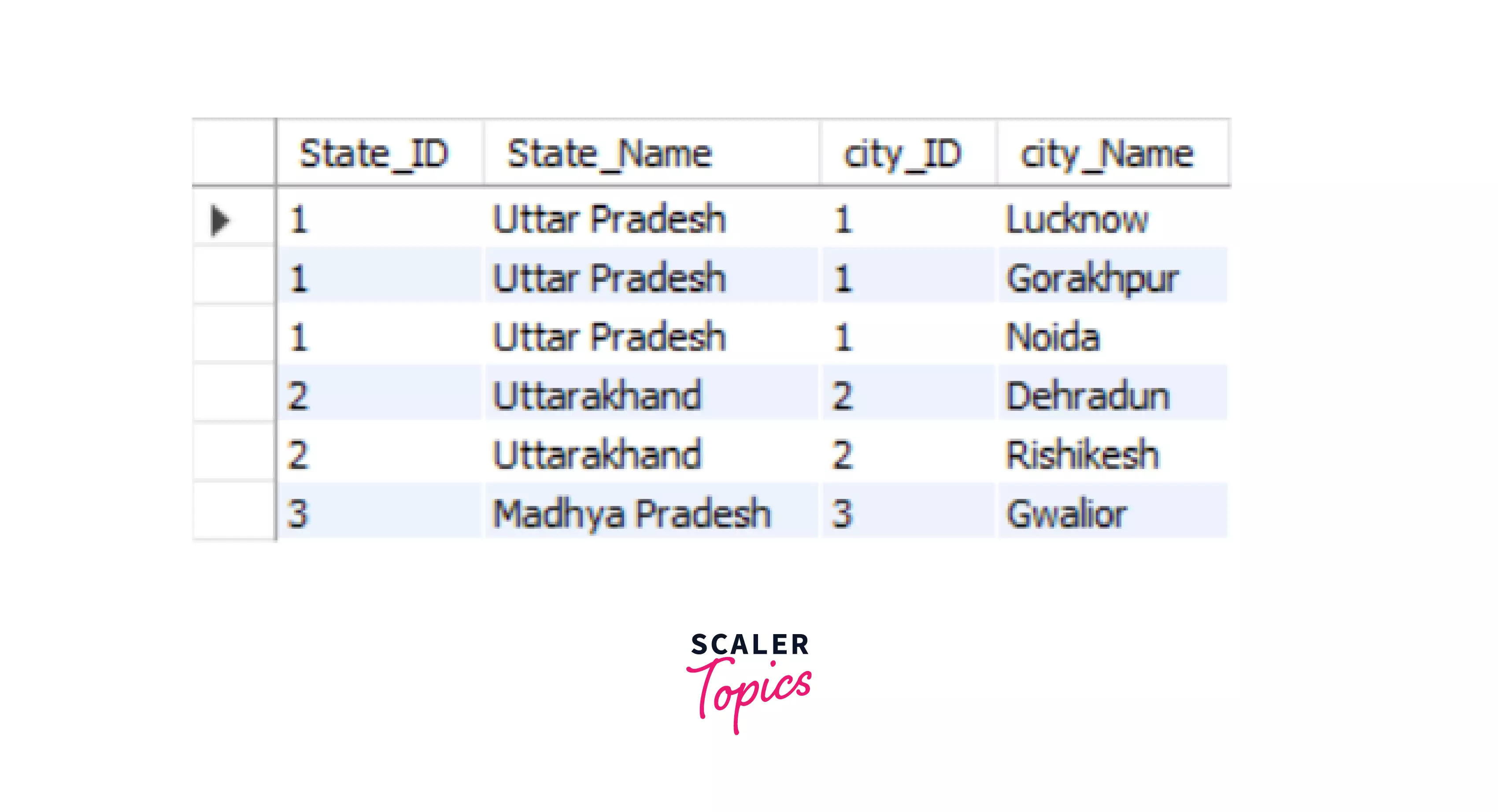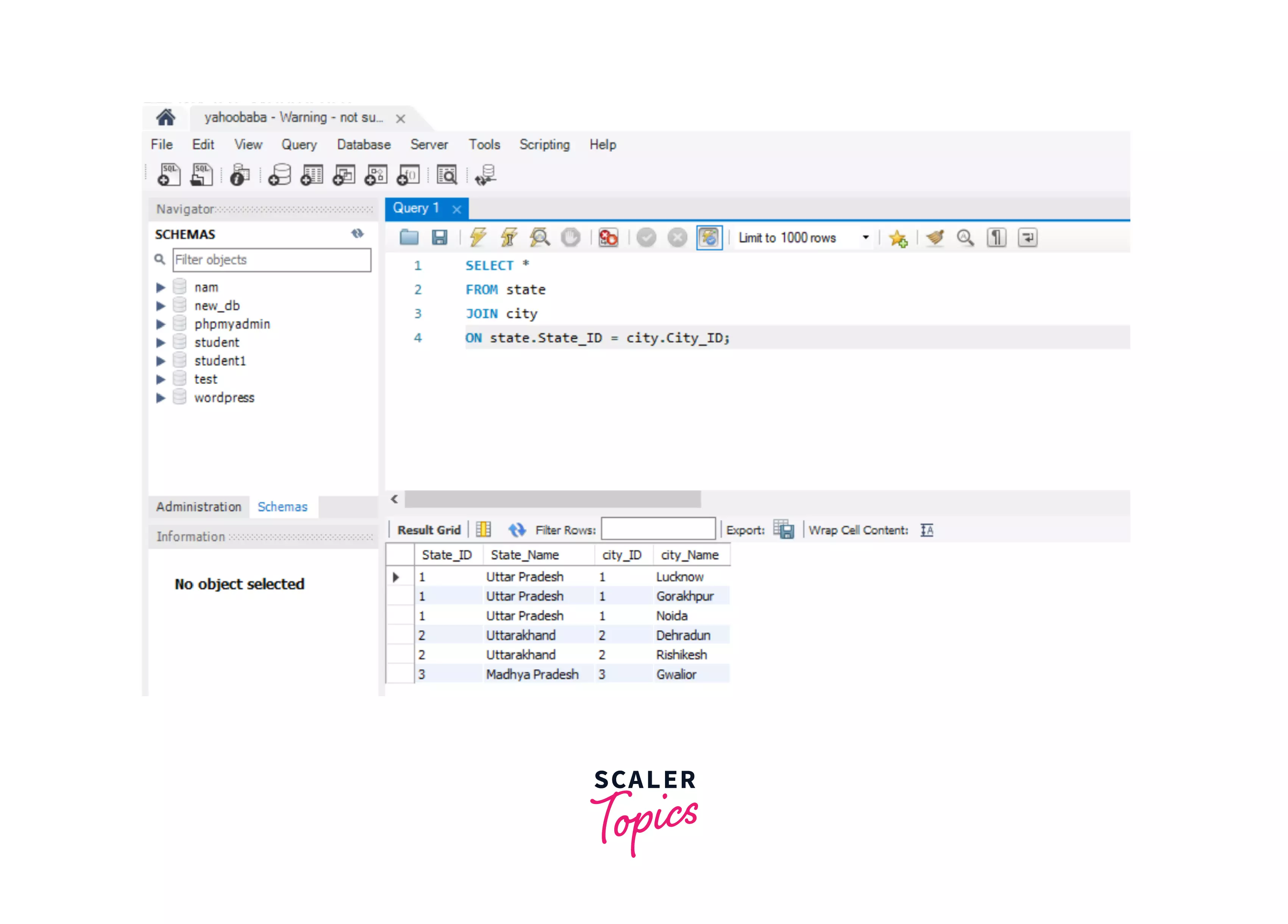# Equi Join and Non-Equi Join in SQL

Challenge Inside! : Find out where you stand! Try quiz, solve problems & win rewards!
Learn via video courseDBMS Course - Master the Fundamentals and Advanced Concepts
By Srikanth VarmaFree
5Enrolled: 1000DBMS Course - Master the Fundamentals and Advanced Concepts
Srikanth VarmaFree
5
Enrolled: 1000

## Overview

An equi join is any JOIN operation that uses only and only the equals sign. If there is a query with more than one join condition, out of which one condition has an equals sign, and the other doesn't, then this query would be considered a non-equi join in SQL. Thus Equi Joins in SQL joins multiple tables on the basis of an equality condition. In contrast, the Non-Equi Join joins the table on the basis of conditions other than the equality conditions, such as !=, >, <, etc.

## Introduction

Whenever we have to retrieve data from a table, we use selection, projection, and join methods. Selection is a method in which we have to retrieve data using a condition such as a WHERE condition from a single table. Projection is a method in which we have to retrieve data from a single table without using a condition. We use joins if we want to select or retrieve data from multiple tables. It retrieves data that includes related rows/data from multiple tables.

Equi and Non-Equi Join in SQL are types of joins that retrieve data from multiple tables based on some condition. Equi Join in SQL will retrieve only the data that is equal in both the tables or only retrieves the matching column values; therefore, it uses an equality operator to join multiple tables. On the other hand, Non-Equi join is used to retrieve data without using the equality operator. However, we can use other operators except the equality one in the non-equi join to join multiple tables.

## EQUI JOIN in SQL

Equi Join in SQL is a type of INNER Join that displays the output by performing a join operation between two or more tables based on the common column between them. It uses the equality ( = ) symbol to compare the data between two columns; if the data matches, it retrieves it. Equi Join compares each column value of the source table with each value in the corresponding target table, and if both the values are equal, it retrieves it.

The Equi Join in SQL returns only the data in all the tables we are comparing based on the common column field. It does not display null or unmatchable data. The equality operator in the Equi Join operation is used to refer to the equality in the WHERE clause. However, it returns the same result when we use the JOIN keyword with the ON clause along with column names and their respective tables.

Syntax:

OR

Example:

Suppose we have two tables, namely state and city, which contain the name of the states and the name of the cities, respectively. In this example, we will map the cities with the states in which they are present.

The table state is shown below:

State_IDState_Name
2Uttarakhand

The table city is shown below:

City_IDCity_Name
1Lucknow
1Gorakhpur
1Noida
2Rishikesh
3Gwalior

Now, if we execute a query of Equi-join using the equality operation and the WHERE clause, then-

Output:We can try the above example with the second syntax-Therefore, we can retrieve the data from two tables using these two methods.

## NON-EQUI JOIN in SQL

Non-Equi Join is also a type of INNER Join in which we need to retrieve data from multiple tables. Non-Equi Join matches the column values from different tables based on an inequality based on the operators like <,>,<=,>=,!=, BETWEEN, etc.

Non-Equi Join in SQL retrieves data using any operator or condition except the equality condition. The value of the column in each row from the source table is compared with the corresponding value of the target table. If the data matches in the source and target table, the comparison returns true, and therefore that data is retrieved from the table.

However, we use the Non-Equi joins for the below-mentioned reasons-

1. Retrieving data matching in a range of values.
2. Checking for duplicate data between tables.
3. For calculating totals.

Syntax:

Example:

1. We take two tables, test1 and test2.

Table test1 as shown below -

S_NOName
20Amit
30Ankush
10Akash
50Jatin

Table test2 is shown below -

S_NOName
80Tarun
60Mitali
10Akash
50Jatin
5Aman

Now, if we execute a query of Non-Equi-join using any operator other than the equality operator, such as >(greater than) with the WHERE clause -

Output:In the above example, we are combining the tables based on their SNo. All those entries will be retrieved whose SNo of the test1 table is greater than the SNo of the test2 table.

## Conclusion

• Joins are used to retrieve data from multiple tables based on a common column.
• Equi Join in SQL and Non-Equi Joins in SQL are types of Inner Joins.
• Equi Join in SQL is used to retrieve data from multiple tables using an equality condition with the WHERE clause.
• Non-Equi in SQL is used to retrieve data from multiple tables using any other operator except the equality condition.
• We can use Non-Equi joins in SQL to check for duplicate data, retrieve data between a range of values, and calculate totals of data or values.
• In both the Equi and Non-Equi joins, each value of the common column of the source table is compared with the value in the target table and based on the equality or inequality conditions present in the query, data will be retrieved from multiple tables.